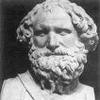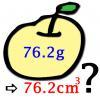#### You may also likeA 1 metre cube has one face on the ground and one face against a wall. A 4 metre ladder leans against the wall and just touches the cube. How high is the top of the ladder above the ground?### Archimedes and Numerical Roots

The problem is how did Archimedes calculate the lengths of the sides of the polygons which needed him to be able to calculate square roots?### More or Less?

Are these estimates of physical quantities accurate?

# Big and Small Numbers in Chemistry

##### Age 14 to 16Challenge Level

1) According to the Royal Mint, a copper coin weighs 3.56g. The relative atomic mass (RAM) is 63.5. The coin therefore contains approximately $\frac{3.56}{63.5}\times6.02\times10^{23} = 3.28\times10^{22}$ copper atoms. Assuming each atom is neutral, each atom contains 29 electrons, so there are $9.52\times10^{23}$ electrons in one coin.

2) I estimated that a lightbulb contains 100ml of air. The mole fraction of argon in dry air is 0.00934. We therefore need $\frac{100}{0.00934}ml = 1.07\times10^4ml = 10.7l$ of air.

3) Assuming the density of air is around 1.2kg/m$^3$, the mass of the air from q2 is $1.2\times10.7\times10^{-3}$kg$= 1.3\times10^{-2}$kg. If this is cooled to a temperature where it's all liquid and it's kept at standard pressure, we can assume it has the standard density of liquid air: 870 kg/m3. It would approximately occupy $\frac{1.3\times10^{-2}}{870}m^3 = 1.5\times10^{-5}m^3 = 0.015l$.

4) The percentage mass of carbon in teflon is $100\times\frac{2\times12}{2\times12+4\times19} = 24\%$. There's an image of the polymer here

5) The number of molecules of the monomer in a chain of length 1cm is $\frac{0.01m}{140pm\times2} = 3.5\times10^7.$ There are therefore $1.4\times10^8$ atoms of fluorine in such a chain.

One mole of $F_2$ gas contains 2 moles of F atoms. The number of moles of fluorine gas is therefore $\frac{ 2.8\times10^8}{2\times(6\times10^{23})} = 2\times10^{-16}$ moles.

6) The reaction is 2M (s) + 2H2O (l)$\rightarrow$ 2MOH (aq) + H2 (g), producing hydrogen gas. To produce 1.5 moles of gas, we'd need 3 moles of water, which weighs 54g and hence has volume 54ml.

7) The nucleus takes up a percentage volume $100\times\frac{\pi\times(1.75fm)^3}{\pi\times(120pm)^3} = 3\times10^{-13}\%$. The rest of the atom is a cloud of negatively charged electrons, which have much, much smaller mass than the nucleus.

8) Given the percentage mass of oxygen of your body is 65%, the percentage mass of carbon of your body is $65\times\frac{9.5}{25.5}\times\frac{12}{16} = 18$%. (This accounts for carbon being less abundant, and also a carbon atom is lighter than an oxygen atom.)

Suppose the average atomic mass is 10gmol$^{-1}$. Then the number of atoms in a human weighing 80kg is $\frac{8\times10^4}{10}\times6\times10^{23} \approx 5\times10^{27}$.

9)A lower bound for this distance is $5\times10^{27}\times120pm = 6\times10^{17}m$ (as hydrogen is the smallest atom). This is about 63 lightyears (the distance light travels in 63 years). There is apparently a masssive planet this distance away.